Next: EXAMPLES, TEMPERATURE FROM GF Up: Laplace and Helmholtz Equation Previous: Steady, 1-D Body, Rectangular

Consider steady heat transfer in any orthogonal coordinate system: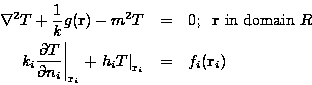where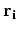is located on surface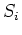. The boundary condition is type 3; heat transfer coefficient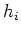can vary with position on surfacebut is independent of temperature. Boundary conditions of type 1 and 2 may be obtained from the above boundary condition can be obtained by setting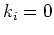or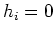, respectively.

The Green's function solution equation for steady temperature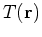is given by: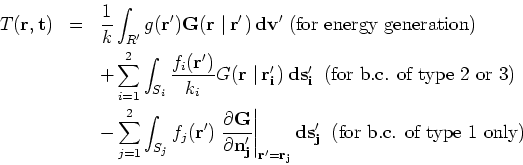See Table 1 (under Heat Equation, General Case) for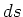and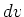for various geometries in rectangular, cylindrical, and spherical coordinates.

2004-01-31